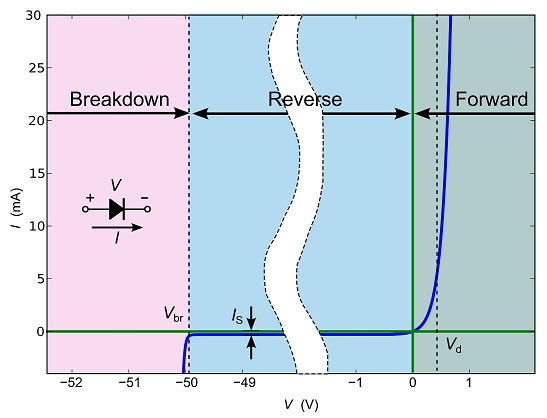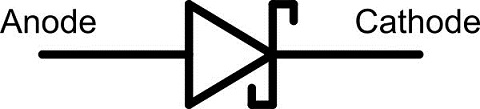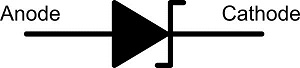# Difference Between Schottky and Zener Diode

## Main Difference – Schottky vs. Zener Diode

Schottky diodes and Zener diodes are two different types of diodes. The main difference between Schottky and Zener diode is that a Schottky diode is made of a metal-semiconductor junction whereas a Zener diode is made of a p-n junction of two highly-doped semiconductors.

## What is a Diode

In electric circuits, a diode is a component that allows the current to flow only in one direction. Typically, a diode is constructed by putting a p-type and an n-type semiconductor in contact. How this structure lets a diode conduct current in one direction is discussed in the article “Difference Between Zener and Avalanche Breakdown“. Essentially, if we draw a graph of how the current through a diode varies as the potential difference across the diode changes, we will get a graph like like the one shown below:Current-Voltage Characteristic for a Diode

## What is a Schottky Diode

A Schottky diode is a special type of diode, constructed using a metal-semiconductor junction instead of a p-n junction used in other diodes. Due to this, the voltage drop across a Schottky diode when it is conducting a forward current (the “cut-in voltage”) is small compared to normal diodes. This is evident in the graph comparing current-voltage characteristics shown below. Note that when the forward voltages are lower, the reverse currents are larger, which is one of the disadvantages for a Schottky diode:The Schottky diodes (blue and green curves) conduct current at much lower forward voltages compared to normal diodes made of p-n junctions.

When a diode conducting a forward current is quickly put under reverse bias or is switched off, it takes a small amount of time for the forward current flowing through the diode to die down. The time taken for this is called reverse recovery time. Compared to normal diodes, the reverse recovery times of Schottky diodes is much smaller, making them suitable to be used in fast switching circuits.

Schottky diodes are used for voltage clamping applications, and in situations where the efficiency of the circuit needs to be maximized (since they have a low potential difference across them, they dissipate less power). For instance, they are used in the construction of solar cells. The circuit symbol for a Schottky diode is shown below:Symbol of a Schottky Diode

## What is a Zener Diode

Zener diodes use a p-n junction just like ordinary diodes. However, Zener diodes are heavily doped compared to normal diodes. As a result, Zener diodes can undergo breakdown without becoming damaged. They also undergo breakdown at a smaller reverse voltage compared to normal diodes, and they maintain this reverse voltage even as they conduct larger reverse currents. Therefore, Zener diodes are useful as voltage regulators in circuits.

The voltage-current characteristic and the circuit symbol of a Zener diode is shown below:Zener Diode Current-Voltage CharacteristicZener Diode Symbol

## Difference Between Schottky and Zener Diode

### Construction

Schottky diode is made of a metal-semiconduction junction

Zener diode is made of a p-n junction between two highly doped semiconductors.

### Reverse Breakdown Voltage

For a Schottky diode, the breakdown voltage is quite high.

For a Zener diode, breakdown occurs at a relatively low reverse voltage.

### Cut-in Voltage

The cut-in voltage for a Schottky diode is comparatively smaller than that for a Zener diode.

For a Zener diode, the cut-in voltage is comparatively higher.

### Reverse Recovery Time

The reverse recovery time for a Schottky diode is very small.

The reverse recovery time for a Zener diode is comparatively longer.

Image Courtesy

“Current vs voltage for a semiconductor diode rectifier” by User:Hldsc(Own work) [CC BY-SA 4.0], via Wikimedia Commons

“Diode-IV-Curve” by Reinraum (Own work) [CC0 1.0], via Wikimedia Commons

“Schematic V-A characteristics of avalanche or Zener diode. (Note: with breakdown voltage over ca. 6 V avalanche diodes are used instead of Zener diodes.)” by Filip Dominec (Own work) [CC BY-SA 3.0], via Wikimedia Commons

“The circuit diagram symbol for a Zener diode. When used in a circuit diagram, the words “Anode” and “Cathode” are not included with the graphic symbol. (Revised to conform to ANSI Y32.2-1975 and IEEE-Std. 315-1975.)” by Omegatron (Own work) [CC BY-SA 3.0], via Wikimedia Commons